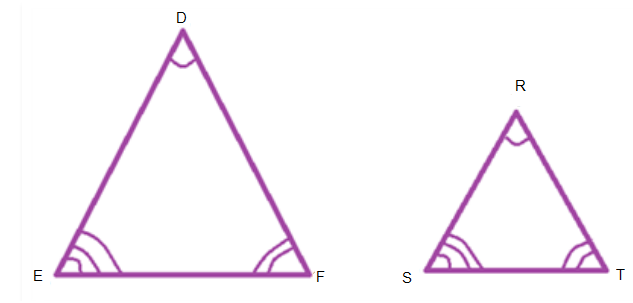# Properties Of Similar Triangles

Two figures are said to be similar if they have the same shape and necessarily not the same size. For example, we can say all circles are similar. All squares are similar and equilateral triangles are similar. All congruent figures are similar but similar figures need not be congruent. In this article, we will learn the properties of similar triangles.

## What are the Properties of Similar Triangles

Property 1:

Two triangles are similar if their corresponding angles are equal and their corresponding sides are within the same ratio (or proportion). Similar triangles will have the same shape, but not necessarily the same size.

Consider two triangles DEF and RST.ΔDEF ~ ΔRST

∠D = ∠R, ∠E = ∠S and ∠F = ∠T

DE/RS = EF/ST = DF/RT.

*The ratio is called the scale factor. The symbol ~ is used to denote similarity.

Property 2:

If corresponding angles of two triangles are equal, then the triangles are similar. They are called equiangular triangles. A famous Greek mathematician Thales gave an important result relating to two equiangular triangles. He used a result called the Basic Proportionality Theorem, which is known as the Thales Theorem.

Example

Given ΔABC ~ ΔXYZ. If AB = 4 cm, BC = 5 cm, AC = 6 cm and XY = 8 cm find YZ and XZ.

Solution:

ΔABC ~ ΔXYZ

So AB/XY = BC/YZ = AC/XZ

4/8 = 5/YZ

YZ = 8×5/4 = 10 cm

4/8 = 6/XZ

XZ = 8×6/4 = 12 cm